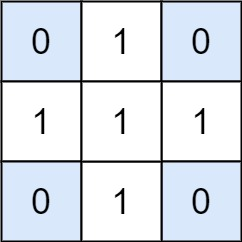# GeetCode Hub

Given a `matrix` and a `target`, return the number of non-empty submatrices that sum to target.

A submatrix `x1, y1, x2, y2` is the set of all cells `matrix[x][y]` with `x1 <= x <= x2` and `y1 <= y <= y2`.

Two submatrices `(x1, y1, x2, y2)` and `(x1', y1', x2', y2')` are different if they have some coordinate that is different: for example, if `x1 != x1'`.

Example 1:```Input: matrix = [[0,1,0],[1,1,1],[0,1,0]], target = 0
Output: 4
Explanation: The four 1x1 submatrices that only contain 0.
```

Example 2:

```Input: matrix = [[1,-1],[-1,1]], target = 0
Output: 5
Explanation: The two 1x2 submatrices, plus the two 2x1 submatrices, plus the 2x2 submatrix.
```

Example 3:

```Input: matrix = [], target = 0
Output: 0
```

Constraints:

• `1 <= matrix.length <= 100`
• `1 <= matrix.length <= 100`
• `-1000 <= matrix[i] <= 1000`
• `-10^8 <= target <= 10^8`

class Solution { public int numSubmatrixSumTarget(int[][] matrix, int target) { } }